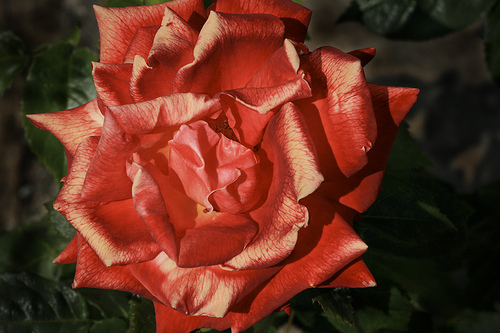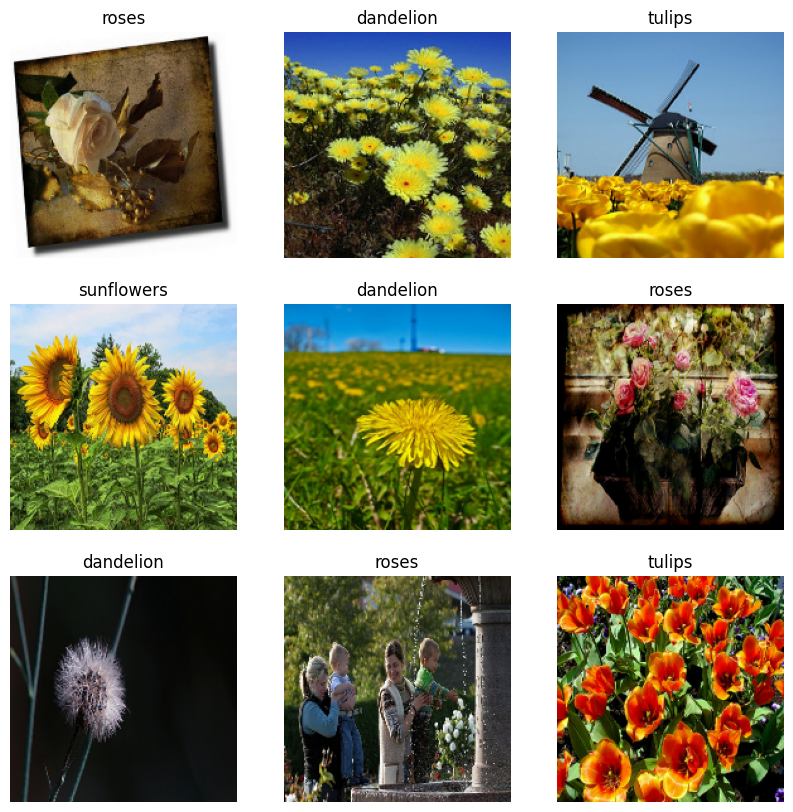This tutorial shows how to load and preprocess an image dataset in two ways. First, you will use high-level Keras preprocessing utilities and layers. Next, you will write your own input pipeline from scratch using tf.data.

## Setup

````pip install -q tf-nightly`
```
``````import numpy as np
import os
import PIL
import PIL.Image
import tensorflow as tf
``````
``````print(tf.__version__)
``````
```2.4.0-dev20200709

```

This tutorial uses a dataset of several thousand photos of flowers. The flowers dataset contains 5 sub-directories, one per class:

``````flowers_photos/
daisy/
dandelion/
roses/
sunflowers/
tulips/
``````
``````import pathlib
data_dir = tf.keras.utils.get_file(origin=dataset_url,
fname='flower_photos',
untar=True)
data_dir = pathlib.Path(data_dir)
``````
```Downloading data from https://storage.googleapis.com/download.tensorflow.org/example_images/flower_photos.tgz
228818944/228813984 [==============================] - 2s 0us/step

```

After downloading (218MB), you should now have a copy of the flower photos available. There are 3670 total images:

``````image_count = len(list(data_dir.glob('*/*.jpg')))
print(image_count)
``````
```3670

```

Each directory contains images of that type of flower. Here are some roses:

``````roses = list(data_dir.glob('roses/*'))
PIL.Image.open(str(roses))
````````````roses = list(data_dir.glob('roses/*'))
PIL.Image.open(str(roses))
``````Let's load these images off disk using image_dataset_from_directory.

## Create a dataset

Define some parameters for the loader:

``````batch_size = 32
img_height = 180
img_width = 180
``````

It's good practice to use a validation split when developing your model. We will use 80% of the images for training, and 20% for validation.

``````train_ds = tf.keras.preprocessing.image_dataset_from_directory(
data_dir,
validation_split=0.2,
subset="training",
seed=123,
image_size=(img_height, img_width),
batch_size=batch_size)
``````
```Found 3670 files belonging to 5 classes.
Using 2936 files for training.

```
``````val_ds = tf.keras.preprocessing.image_dataset_from_directory(
data_dir,
validation_split=0.2,
subset="validation",
seed=123,
image_size=(img_height, img_width),
batch_size=batch_size)
``````
```Found 3670 files belonging to 5 classes.
Using 734 files for validation.

```

You can find the class names in the `class_names` attribute on these datasets.

``````class_names = train_ds.class_names
print(class_names)
``````
```['daisy', 'dandelion', 'roses', 'sunflowers', 'tulips']

```

## Visualize the data

Here are the first 9 images from the training dataset.

``````import matplotlib.pyplot as plt

plt.figure(figsize=(10, 10))
for images, labels in train_ds.take(1):
for i in range(9):
ax = plt.subplot(3, 3, i + 1)
plt.imshow(images[i].numpy().astype("uint8"))
plt.title(class_names[labels[i]])
plt.axis("off")
``````You can train a model using these datasets by passing them to `model.fit` (shown later in this tutorial). If you like, you can also manually iterate over the dataset and retrieve batches of images:

``````for image_batch, labels_batch in train_ds:
print(image_batch.shape)
print(labels_batch.shape)
break
``````
```(32, 180, 180, 3)
(32,)

```

The `image_batch` is a tensor of the shape `(32, 180, 180, 3)`. This is a batch of 32 images of shape `180x180x3` (the last dimension referes to color channels RGB). The `label_batch` is a tensor of the shape `(32,)`, these are corresponding labels to the 32 images.

## Standardize the data

The RGB channel values are in the `[0, 255]` range. This is not ideal for a neural network; in general you should seek to make your input values small. Here, we will standardize values to be in the `[0, 1]` by using a Rescaling layer.

``````from tensorflow.keras import layers

normalization_layer = tf.keras.layers.experimental.preprocessing.Rescaling(1./255)
``````

There are two ways to use this layer. You can apply it to the dataset by calling map:

``````normalized_ds = train_ds.map(lambda x, y: (normalization_layer(x), y))
image_batch, labels_batch = next(iter(normalized_ds))
first_image = image_batch
# Notice the pixels values are now in `[0,1]`.
print(np.min(first_image), np.max(first_image))
``````
```WARNING:tensorflow:AutoGraph could not transform <function <lambda> at 0x7f3ff2cc6a60> and will run it as-is.
Please report this to the TensorFlow team. When filing the bug, set the verbosity to 10 (on Linux, `export AUTOGRAPH_VERBOSITY=10`) and attach the full output.
Cause: <module '__main__'> is a built-in module
To silence this warning, decorate the function with @tf.autograph.experimental.do_not_convert
WARNING: AutoGraph could not transform <function <lambda> at 0x7f3ff2cc6a60> and will run it as-is.
Please report this to the TensorFlow team. When filing the bug, set the verbosity to 10 (on Linux, `export AUTOGRAPH_VERBOSITY=10`) and attach the full output.
Cause: <module '__main__'> is a built-in module
To silence this warning, decorate the function with @tf.autograph.experimental.do_not_convert
0.0 0.96902645

```

Or, you can include the layer inside your model definition to simplify deployment. We will use the second approach here.

## Configure the dataset for performance

Let's make sure to use buffered prefetching so we can yield data from disk without having I/O become blocking. These are two important methods you should use when loading data.

`.cache()` keeps the images in memory after they're loaded off disk during the first epoch. This will ensure the dataset does not become a bottleneck while training your model. If your dataset is too large to fit into memory, you can also use this method to create a performant on-disk cache.

`.prefetch()` overlaps data preprocessing and model execution while training.

``````AUTOTUNE = tf.data.experimental.AUTOTUNE

train_ds = train_ds.cache().prefetch(buffer_size=AUTOTUNE)
val_ds = val_ds.cache().prefetch(buffer_size=AUTOTUNE)
``````

## Train a model

For completeness, we will show how to train a simple model using the datasets we just prepared. This model has not been tuned in any way - the goal is to show you the mechanics using the datasets you just created. To learn more about image classification, visit this tutorial.

``````num_classes = 5

model = tf.keras.Sequential([
layers.experimental.preprocessing.Rescaling(1./255),
layers.Conv2D(32, 3, activation='relu'),
layers.MaxPooling2D(),
layers.Conv2D(32, 3, activation='relu'),
layers.MaxPooling2D(),
layers.Conv2D(32, 3, activation='relu'),
layers.MaxPooling2D(),
layers.Flatten(),
layers.Dense(128, activation='relu'),
layers.Dense(num_classes)
])
``````
``````model.compile(
loss=tf.losses.SparseCategoricalCrossentropy(from_logits=True),
metrics=['accuracy'])
``````
``````model.fit(
train_ds,
batch_size=batch_size,
validation_data=val_ds,
epochs=3
)
``````
```Epoch 1/3
92/92 [==============================] - 7s 74ms/step - loss: 1.3345 - accuracy: 0.4131 - val_loss: 1.0854 - val_accuracy: 0.5845
Epoch 2/3
92/92 [==============================] - 1s 11ms/step - loss: 1.0263 - accuracy: 0.5909 - val_loss: 1.0316 - val_accuracy: 0.5886
Epoch 3/3
92/92 [==============================] - 1s 11ms/step - loss: 0.8839 - accuracy: 0.6574 - val_loss: 0.9057 - val_accuracy: 0.6431

<tensorflow.python.keras.callbacks.History at 0x7f3fb027a5c0>
```

You may notice the validation accuracy is low to the compared to the training accuracy, indicating our model is overfitting. You can learn more about overfitting and how to reduce it in this tutorial.

# Using tf.data for finer control

The above keras.preprocessing utilities are a convenient way to create a `tf.data.Dataset` from a directory of imgages. For finer grain control, you can write your own input pipeline using `tf.data`. This section shows how to do just that, beginning with the file paths from the zip we downloaded earlier.

``````list_ds = tf.data.Dataset.list_files(str(data_dir/'*/*'))
list_ds = list_ds.shuffle(image_count, reshuffle_each_iteration=False)
``````
``````for f in list_ds.take(5):
print(f.numpy())
``````
```b'/home/kbuilder/.keras/datasets/flower_photos/tulips/4574785121_5d8ec4626e.jpg'
b'/home/kbuilder/.keras/datasets/flower_photos/roses/537207677_f96a0507bb.jpg'
b'/home/kbuilder/.keras/datasets/flower_photos/tulips/3990746027_338ee436d2_n.jpg'
b'/home/kbuilder/.keras/datasets/flower_photos/tulips/14087860553_bf4f8ec56d.jpg'
b'/home/kbuilder/.keras/datasets/flower_photos/dandelion/5110102140_787d325757_n.jpg'

```

The tree structure of the files can be used to compile a `class_names` list.

``````class_names = np.array(sorted([item.name for item in data_dir.glob('*') if item.name != "LICENSE.txt"]))
print(class_names)
``````
```['daisy' 'dandelion' 'roses' 'sunflowers' 'tulips']

```

Split the dataset into train and validation:

``````val_size = int(image_count * 0.2)
train_ds = list_ds.skip(val_size)
val_ds = list_ds.take(val_size)
``````

You can see the length of each dataset as follows:

``````print(tf.data.experimental.cardinality(train_ds).numpy())
print(tf.data.experimental.cardinality(val_ds).numpy())
``````
```2936
734

```

Write a short function that converts a file path to an `(img, label)` pair:

``````def get_label(file_path):
# convert the path to a list of path components
parts = tf.strings.split(file_path, os.path.sep)
# The second to last is the class-directory
one_hot = parts[-2] == class_names
# Integer encode the label
return tf.argmax(one_hot)
``````
``````def decode_img(img):
# convert the compressed string to a 3D uint8 tensor
img = tf.image.decode_jpeg(img, channels=3)
# resize the image to the desired size
return tf.image.resize(img, [img_height, img_width])
``````
``````def process_path(file_path):
label = get_label(file_path)
# load the raw data from the file as a string
img = decode_img(img)
return img, label
``````

Use `Dataset.map` to create a dataset of `image, label` pairs:

``````# Set `num_parallel_calls` so multiple images are loaded/processed in parallel.
train_ds = train_ds.map(process_path, num_parallel_calls=AUTOTUNE)
val_ds = val_ds.map(process_path, num_parallel_calls=AUTOTUNE)
``````
``````for image, label in train_ds.take(1):
print("Image shape: ", image.numpy().shape)
print("Label: ", label.numpy())
``````
```Image shape:  (180, 180, 3)
Label:  3

```

## Configure dataset for performance

To train a model with this dataset you will want the data:

• To be well shuffled.
• To be batched.
• Batches to be available as soon as possible.

These features can be added using the `tf.data` API. For more details, see the Input Pipeline Performance guide.

``````def configure_for_performance(ds):
ds = ds.cache()
ds = ds.shuffle(buffer_size=1000)
ds = ds.batch(batch_size)
ds = ds.prefetch(buffer_size=AUTOTUNE)
return ds

train_ds = configure_for_performance(train_ds)
val_ds = configure_for_performance(val_ds)
``````

## Visualize the data

You can visualize this dataset similarly to the one you created previously.

``````image_batch, label_batch = next(iter(train_ds))

plt.figure(figsize=(10, 10))
for i in range(9):
ax = plt.subplot(3, 3, i + 1)
plt.imshow(image_batch[i].numpy().astype("uint8"))
label = label_batch[i]
plt.title(class_names[label])
plt.axis("off")
``````## Continue training the model

You have now manually built a similar `tf.data.Dataset` to the one created by the `keras.preprocessing` above. You can continue training the model with it. As before, we will train for just a few epochs to keep the running time short.

``````model.fit(
train_ds,
validation_data=val_ds,
epochs=3
)
``````
```Epoch 1/3
92/92 [==============================] - 2s 21ms/step - loss: 0.7307 - accuracy: 0.7245 - val_loss: 0.5721 - val_accuracy: 0.7779
Epoch 2/3
92/92 [==============================] - 1s 12ms/step - loss: 0.5434 - accuracy: 0.7984 - val_loss: 0.4317 - val_accuracy: 0.8610
Epoch 3/3
92/92 [==============================] - 1s 11ms/step - loss: 0.3687 - accuracy: 0.8668 - val_loss: 0.3982 - val_accuracy: 0.8665

<tensorflow.python.keras.callbacks.History at 0x7f40512597b8>
```

# Next steps

This tutorial showed two ways of loading images off disk. First, you learned how to load and preprocess an image dataset using Keras preprocessing layers and utilities. Next, you learned how to write an input pipeline from scratch using tf.data. As a next step, you can learn how to add data augmentation by visiting this tutorial. To learn more about tf.data, you can visit this guide.